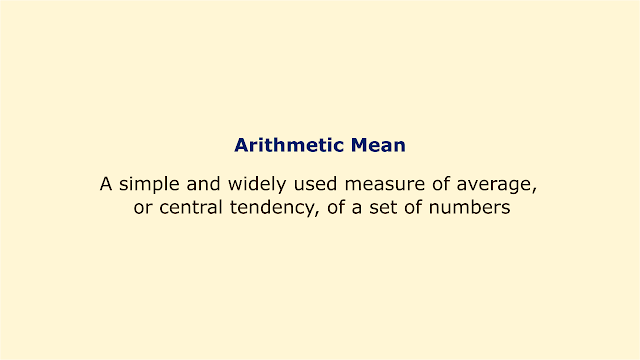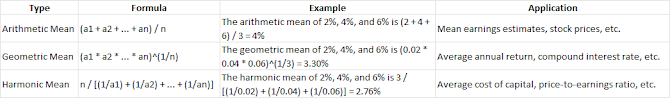# Arithmetic MeanImage: Moneybestpal.com

### The arithmetic mean is an easy and popular way to calculate the average or central tendency of a group of integers. It is calculated by dividing the total number of numbers by their count. For instance, (2 + 4 + 6 + 8) / 4 = 5 is the arithmetic mean of 2, 4, 6, and 8.

Arithmetic means are frequently used in finance to predict future values based on historical data, such as stock prices, earnings, dividends, etc. However, it is not always the best strategy to determine an average, particularly when there are outliers or compounding effects that can greatly affect the mean.

In finance, geometric mean and harmonic mean are two other types of means that are more frequently utilized. Calculating the average yearly return on a compounding investment is frequently done using the geometric mean, which is the nth root of the product of n values. The average cost of capital or price-to-earnings ratio are frequently determined using the harmonic mean, which is the reciprocal of the arithmetic mean of the reciprocals of the numbers.

The following table summarizes some of the differences between arithmetic mean, geometric mean, and harmonic mean:Image: Moneybestpal.com

As you can see, the harmonic mean and geometric mean are always greater than or equal to each other, and the arithmetic mean is always greater than or equal to each of them.

When determining the average or central tendency of a group of numbers in finance, the arithmetic mean has certain drawbacks and alternatives. It is still a practical and straightforward instrument, nevertheless, that can offer insightful analysis and projections for money.
Tags